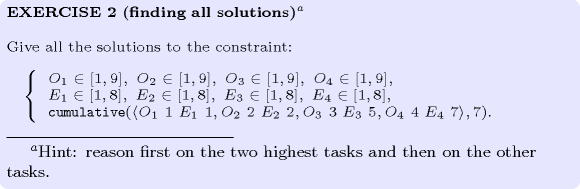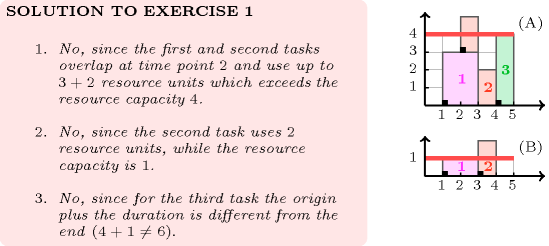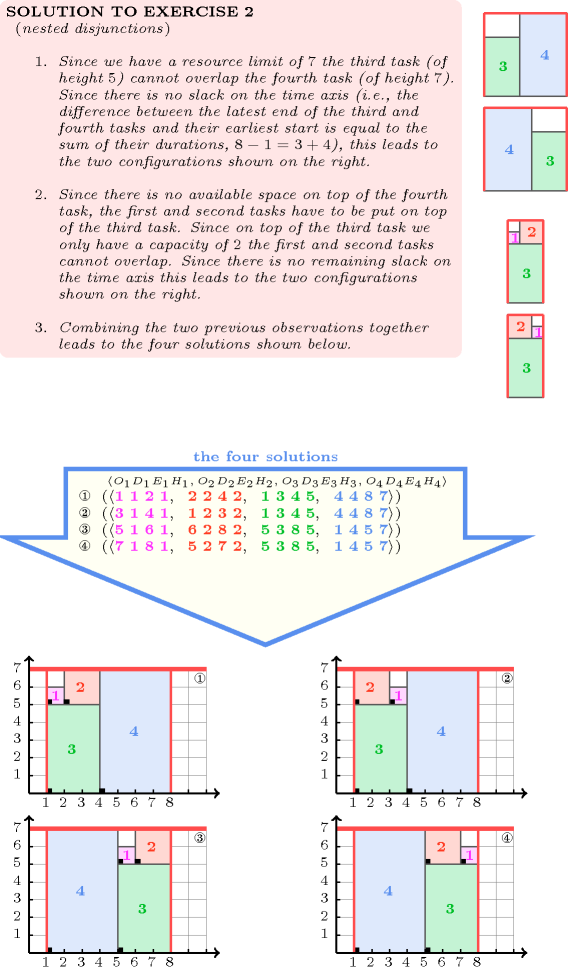## 5.96. cumulative

Origin
Constraint

$\mathrm{𝚌𝚞𝚖𝚞𝚕𝚊𝚝𝚒𝚟𝚎}\left(\mathrm{𝚃𝙰𝚂𝙺𝚂},\mathrm{𝙻𝙸𝙼𝙸𝚃}\right)$

Synonym

$\mathrm{𝚌𝚞𝚖𝚞𝚕𝚊𝚝𝚒𝚟𝚎}_\mathrm{𝚖𝚊𝚡}$.

Arguments
 $\mathrm{𝚃𝙰𝚂𝙺𝚂}$ $\mathrm{𝚌𝚘𝚕𝚕𝚎𝚌𝚝𝚒𝚘𝚗}\left(\begin{array}{c}\mathrm{𝚘𝚛𝚒𝚐𝚒𝚗}-\mathrm{𝚍𝚟𝚊𝚛},\hfill \\ \mathrm{𝚍𝚞𝚛𝚊𝚝𝚒𝚘𝚗}-\mathrm{𝚍𝚟𝚊𝚛},\hfill \\ \mathrm{𝚎𝚗𝚍}-\mathrm{𝚍𝚟𝚊𝚛},\hfill \\ \mathrm{𝚑𝚎𝚒𝚐𝚑𝚝}-\mathrm{𝚍𝚟𝚊𝚛}\hfill \end{array}\right)$ $\mathrm{𝙻𝙸𝙼𝙸𝚃}$ $\mathrm{𝚒𝚗𝚝}$
Restrictions
 $\mathrm{𝚛𝚎𝚚𝚞𝚒𝚛𝚎}_\mathrm{𝚊𝚝}_\mathrm{𝚕𝚎𝚊𝚜𝚝}$$\left(2,\mathrm{𝚃𝙰𝚂𝙺𝚂},\left[\mathrm{𝚘𝚛𝚒𝚐𝚒𝚗},\mathrm{𝚍𝚞𝚛𝚊𝚝𝚒𝚘𝚗},\mathrm{𝚎𝚗𝚍}\right]\right)$ $\mathrm{𝚛𝚎𝚚𝚞𝚒𝚛𝚎𝚍}$$\left(\mathrm{𝚃𝙰𝚂𝙺𝚂},\mathrm{𝚑𝚎𝚒𝚐𝚑𝚝}\right)$ $\mathrm{𝚃𝙰𝚂𝙺𝚂}.\mathrm{𝚍𝚞𝚛𝚊𝚝𝚒𝚘𝚗}\ge 0$ $\mathrm{𝚃𝙰𝚂𝙺𝚂}.\mathrm{𝚘𝚛𝚒𝚐𝚒𝚗}\le \mathrm{𝚃𝙰𝚂𝙺𝚂}.\mathrm{𝚎𝚗𝚍}$ $\mathrm{𝚃𝙰𝚂𝙺𝚂}.\mathrm{𝚑𝚎𝚒𝚐𝚑𝚝}\ge 0$ $\mathrm{𝙻𝙸𝙼𝙸𝚃}\ge 0$
Purpose

Cumulative scheduling constraint or scheduling under resource constraints. Consider a set $𝒯$ of tasks described by the $\mathrm{𝚃𝙰𝚂𝙺𝚂}$ collection. The $\mathrm{𝚌𝚞𝚖𝚞𝚕𝚊𝚝𝚒𝚟𝚎}$ constraint enforces that at each point in time, the cumulated height of the set of tasks that overlap that point, does not exceed a given limit. A task overlaps a point $i$ if and only if (1) its origin is less than or equal to $i$, and (2) its end is strictly greater than $i$. It also imposes for each task of $𝒯$ the constraint $\mathrm{𝚘𝚛𝚒𝚐𝚒𝚗}+\mathrm{𝚍𝚞𝚛𝚊𝚝𝚒𝚘𝚗}=\mathrm{𝚎𝚗𝚍}$.

Example
$\left(\begin{array}{c}〈\begin{array}{cccc}\mathrm{𝚘𝚛𝚒𝚐𝚒𝚗}-1\hfill & \mathrm{𝚍𝚞𝚛𝚊𝚝𝚒𝚘𝚗}-3\hfill & \mathrm{𝚎𝚗𝚍}-4\hfill & \mathrm{𝚑𝚎𝚒𝚐𝚑𝚝}-1,\hfill \\ \mathrm{𝚘𝚛𝚒𝚐𝚒𝚗}-2\hfill & \mathrm{𝚍𝚞𝚛𝚊𝚝𝚒𝚘𝚗}-9\hfill & \mathrm{𝚎𝚗𝚍}-11\hfill & \mathrm{𝚑𝚎𝚒𝚐𝚑𝚝}-2,\hfill \\ \mathrm{𝚘𝚛𝚒𝚐𝚒𝚗}-3\hfill & \mathrm{𝚍𝚞𝚛𝚊𝚝𝚒𝚘𝚗}-10\hfill & \mathrm{𝚎𝚗𝚍}-13\hfill & \mathrm{𝚑𝚎𝚒𝚐𝚑𝚝}-1,\hfill \\ \mathrm{𝚘𝚛𝚒𝚐𝚒𝚗}-6\hfill & \mathrm{𝚍𝚞𝚛𝚊𝚝𝚒𝚘𝚗}-6\hfill & \mathrm{𝚎𝚗𝚍}-12\hfill & \mathrm{𝚑𝚎𝚒𝚐𝚑𝚝}-1,\hfill \\ \mathrm{𝚘𝚛𝚒𝚐𝚒𝚗}-7\hfill & \mathrm{𝚍𝚞𝚛𝚊𝚝𝚒𝚘𝚗}-2\hfill & \mathrm{𝚎𝚗𝚍}-9\hfill & \mathrm{𝚑𝚎𝚒𝚐𝚑𝚝}-3\hfill \end{array}〉,8\hfill \end{array}\right)$
##### Figure 5.96.1. Resource consumption profile corresponding to the five tasks of the Example slot (note that the vertical position of a task does not really matter but is only used for displaying the contribution of a task to the resource consumption profile)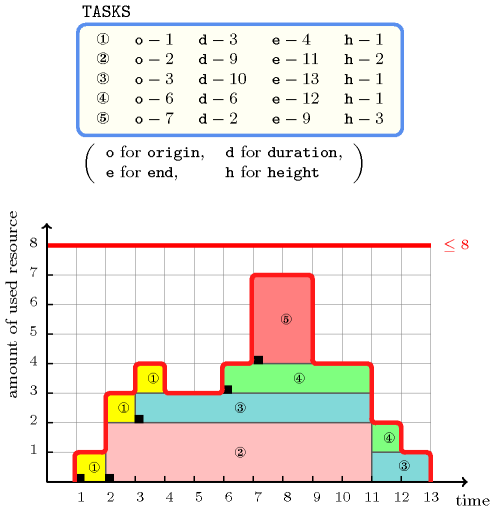Figure 5.96.1 shows the cumulated profile associated with the example. To each task of the $\mathrm{𝚌𝚞𝚖𝚞𝚕𝚊𝚝𝚒𝚟𝚎}$ constraint, i.e. each line of the example, corresponds a set of rectangles coloured with the same colour: the sum of the lengths of the rectangles corresponds to the duration of the task, while the height of the rectangles (i.e., all the rectangles associated with a task have the same height) corresponds to the resource consumption of the task. The $\mathrm{𝚌𝚞𝚖𝚞𝚕𝚊𝚝𝚒𝚟𝚎}$ constraint holds since at each point in time we do not have a cumulated resource consumption strictly greater than the upper limit 8 enforced by the last argument of the $\mathrm{𝚌𝚞𝚖𝚞𝚕𝚊𝚝𝚒𝚟𝚎}$ constraint.

All solutions

Figure 5.96.2 gives all solutions to the following non ground instance of the $\mathrm{𝚌𝚞𝚖𝚞𝚕𝚊𝚝𝚒𝚟𝚎}$ constraint:

${O}_{1}\in \left[1,5\right]$, ${D}_{1}\in \left[4,4\right]$, ${E}_{1}\in \left[1,9\right]$, ${H}_{1}\in \left[2,6\right]$,

${O}_{2}\in \left[2,7\right]$, ${D}_{2}\in \left[6,6\right]$, ${E}_{2}\in \left[1,9\right]$, ${H}_{2}\in \left[3,3\right]$,

${O}_{3}\in \left[3,6\right]$, ${D}_{3}\in \left[3,6\right]$, ${E}_{3}\in \left[1,9\right]$, ${H}_{3}\in \left[1,2\right]$,

${O}_{4}\in \left[1,8\right]$, ${D}_{4}\in \left[2,3\right]$, ${E}_{4}\in \left[1,9\right]$, ${H}_{4}\in \left[3,4\right]$,

$\mathrm{𝚌𝚞𝚖𝚞𝚕𝚊𝚝𝚒𝚟𝚎}$$\left(〈{O}_{1}{D}_{1}{E}_{1}{H}_{1}1,{O}_{2}{D}_{2}{E}_{2}{H}_{2}2,{O}_{3}{D}_{3}{E}_{3}{H}_{3}3,{O}_{4}{D}_{4}{E}_{4}{H}_{4}4〉,5\right)$.

##### Figure 5.96.2. All solutions corresponding to the non ground example of the $\mathrm{𝚌𝚞𝚖𝚞𝚕𝚊𝚝𝚒𝚟𝚎}$ constraint of the All solutions slot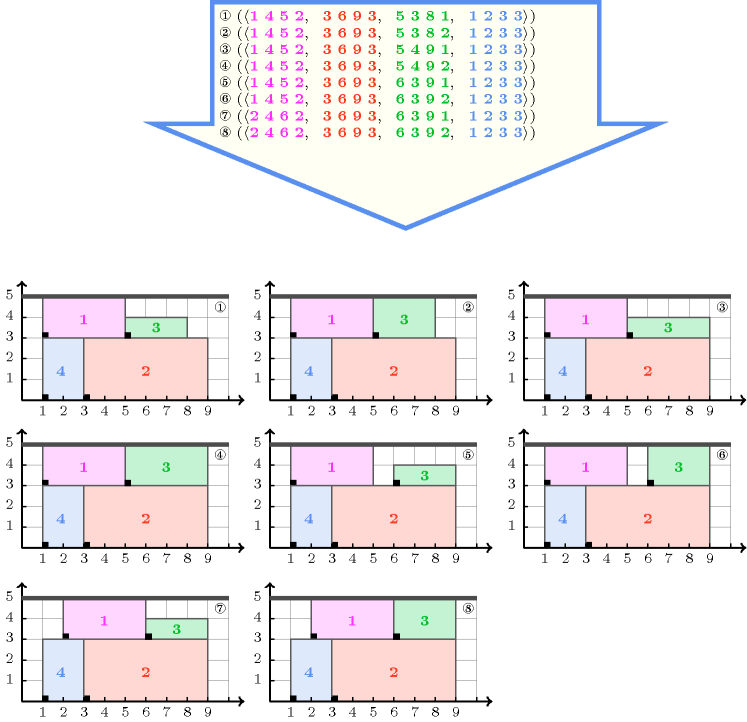Typical
 $|\mathrm{𝚃𝙰𝚂𝙺𝚂}|>1$ $\mathrm{𝚛𝚊𝚗𝚐𝚎}$$\left(\mathrm{𝚃𝙰𝚂𝙺𝚂}.\mathrm{𝚘𝚛𝚒𝚐𝚒𝚗}\right)>1$ $\mathrm{𝚛𝚊𝚗𝚐𝚎}$$\left(\mathrm{𝚃𝙰𝚂𝙺𝚂}.\mathrm{𝚍𝚞𝚛𝚊𝚝𝚒𝚘𝚗}\right)>1$ $\mathrm{𝚛𝚊𝚗𝚐𝚎}$$\left(\mathrm{𝚃𝙰𝚂𝙺𝚂}.\mathrm{𝚎𝚗𝚍}\right)>1$ $\mathrm{𝚛𝚊𝚗𝚐𝚎}$$\left(\mathrm{𝚃𝙰𝚂𝙺𝚂}.\mathrm{𝚑𝚎𝚒𝚐𝚑𝚝}\right)>1$ $\mathrm{𝚃𝙰𝚂𝙺𝚂}.\mathrm{𝚍𝚞𝚛𝚊𝚝𝚒𝚘𝚗}>0$ $\mathrm{𝚃𝙰𝚂𝙺𝚂}.\mathrm{𝚑𝚎𝚒𝚐𝚑𝚝}>0$ $\mathrm{𝙻𝙸𝙼𝙸𝚃}<$$\mathrm{𝚜𝚞𝚖}$$\left(\mathrm{𝚃𝙰𝚂𝙺𝚂}.\mathrm{𝚑𝚎𝚒𝚐𝚑𝚝}\right)$
Symmetries
• Items of $\mathrm{𝚃𝙰𝚂𝙺𝚂}$ are permutable.

• $\mathrm{𝚃𝙰𝚂𝙺𝚂}.\mathrm{𝚍𝚞𝚛𝚊𝚝𝚒𝚘𝚗}$ can be decreased to any value $\ge 0$.

• $\mathrm{𝚃𝙰𝚂𝙺𝚂}.\mathrm{𝚑𝚎𝚒𝚐𝚑𝚝}$ can be decreased to any value $\ge 0$.

• One and the same constant can be added to the $\mathrm{𝚘𝚛𝚒𝚐𝚒𝚗}$ and $\mathrm{𝚎𝚗𝚍}$ attributes of all items of $\mathrm{𝚃𝙰𝚂𝙺𝚂}$.

• $\mathrm{𝙻𝙸𝙼𝙸𝚃}$ can be increased.

Arg. properties

Contractible wrt. $\mathrm{𝚃𝙰𝚂𝙺𝚂}$.

Usage

The $\mathrm{𝚌𝚞𝚖𝚞𝚕𝚊𝚝𝚒𝚟𝚎}$ constraint occurs in most resource scheduling problems where one has to deal with renewable and/or non-renewable resources:

• Renewable resources typically correspond to machines or persons, and tasks require such resources during all their execution (i.e. a resource starts to be used at the beginning of the task and is released at the end of the task). This means that, once a task has finished its work, the resource it was using is available for other tasks. Tasks are defined by their origin, duration, end and resource consumption and can not be interrupted. When the duration and resource consumption are not fixed tasks can be defined by their load, i.e. the product of their duration and resource consumption. To express the dependency between a non-fixed duration/resource consumption of a task with another decision variable (e.g., to state that the duration of a task depends on its start) one can use the $\mathrm{𝚎𝚕𝚎𝚖𝚎𝚗𝚝}$ constraint where the decision variable corresponds to the index argument of the $\mathrm{𝚎𝚕𝚎𝚖𝚎𝚗𝚝}$ constraint.

• Non renewable resources typically correspond to stock or money, i.e., resources that do not come back when a task finishes to use them. In this context the $\mathrm{𝚌𝚞𝚖𝚞𝚕𝚊𝚝𝚒𝚟𝚎}$ constraint is used for modelling producer-consumer problems, i.e. problems where a first set of tasks produces a non-renewable resource, while a second set of tasks consumes this resource so that a limit on the minimum or the maximum stock at each instant is imposed.

The $\mathrm{𝚌𝚞𝚖𝚞𝚕𝚊𝚝𝚒𝚟𝚎}$ constraint is also used as a necessary condition for non-overlapping rectangles (see the $\mathrm{𝚍𝚒𝚏𝚏𝚗}$ constraint).

Remark

In the original $\mathrm{𝚌𝚞𝚖𝚞𝚕𝚊𝚝𝚒𝚟𝚎}$ constraint of CHIP the $\mathrm{𝙻𝙸𝙼𝙸𝚃}$ parameter was a domain variable corresponding to the maximum peak of the resource consumption profile. Given a fixed time frame, this variable could be used as a cost in order to directly minimise the maximum resource consumption peak. Fixing this variable is potentially dangerous since it imposes the maximum peak to be equal to a given target value.

Some systems like Ilog CP Optimizer also assume that a zero-duration task overlaps a point $i$ if and only if (1) its origin is less than or equal to $i$, and (2) its end is greater than or equal to $i$. Under this definition, the height of a zero-duration task is also taken into account in the resource consumption profile.

Note that the concept of cumulative is different from the concept of rectangles non-overlapping even though, most of the time, each task of a ground solution to a $\mathrm{𝚌𝚞𝚖𝚞𝚕𝚊𝚝𝚒𝚟𝚎}$ constraint is simply drawn as a single rectangle. As illustrated by Figure 5.118.7, this is in fact not always possible (i.e., some rectangles may need to be broken apart). In fact the $\mathrm{𝚌𝚞𝚖𝚞𝚕𝚊𝚝𝚒𝚟𝚎}$ constraint is only a necessary condition for rectangles non-overlapping (see Figure 5.118.6 and the corresponding explanation in the Algorithm slot of the $\mathrm{𝚍𝚒𝚏𝚏𝚗}$ constraint).

In MiniZinc (http://www.minizinc.org/) the tasks of a $\mathrm{𝚌𝚞𝚖𝚞𝚕𝚊𝚝𝚒𝚟𝚎}$ constraint have no $\mathrm{𝚎𝚗𝚍}$ attribute.

Algorithm

The first filtering algorithms were related to the notion of compulsory part of a task [Lahrichi82]. They compute a cumulated resource profile of all the compulsory parts of the tasks and prune the origins of the tasks with respect to this profile in order to not exceed the resource capacity. These methods are sometimes called time tabling. Even though these methods are quite local, i.e., a task has a non-empty compulsory part only when the difference between its latest start and its earliest start is strictly less than its duration, it scales well and is therefore widely used. Later on, more global algorithmsEven though these more global algorithms usually can prune more early in the search tree, these algorithms do not catch all deductions derived from the cumulated resource profile of compulsory parts. based on the resource consumption of the tasks on specific intervals were introduced [ErschlerLopez90], [CaseauLaburthe96b], [Lock96]. A popular variant, called edge finding, considers only specific intervals [MercierVanHentenryck08]. An efficient implementation of edge finding in $O\left(knlogn\right)$, where $k$ is the number of distinct task heights and $n$ is the number of tasks, based on a specific data structure, so called a cumulative $\Phi$-tree [Vilim09a], is provided in [Vilim09b]. When the number of distinct task heights $k$ is not small, a usually almost faster implementation in $O\left({n}^{2}\right)$ is described in [KameugneFotsoScottNgoKateu11]. A $O\left({n}^{2}logn\right)$ filtering algorithm based on tasks that can not be the earliest (or not be the latest) is described in [SchuttWolf10].

Within the context of linear programming, the reference [HookerYan02] provides a relaxation of the $\mathrm{𝚌𝚞𝚖𝚞𝚕𝚊𝚝𝚒𝚟𝚎}$ constraint.

A necessary condition for the $\mathrm{𝚌𝚞𝚖𝚞𝚕𝚊𝚝𝚒𝚟𝚎}$ constraint is obtained by stating a $\mathrm{𝚍𝚒𝚜𝚓𝚞𝚗𝚌𝚝𝚒𝚟𝚎}$ constraint on a subset of tasks $𝒯$ such that, for each pair of tasks of $𝒯$, the sum of the two corresponding minimum heights is strictly greater than $\mathrm{𝙻𝙸𝙼𝙸𝚃}$. This can be done by applying the following procedure:

• Let $h$ be the smallest minimum height strictly greater than $⌊\frac{\mathrm{𝙻𝙸𝙼𝙸𝚃}}{2}⌋$ of the tasks of the $\mathrm{𝚌𝚞𝚖𝚞𝚕𝚊𝚝𝚒𝚟𝚎}$ constraint. If no such task exists then the procedure is stopped without stating any $\mathrm{𝚍𝚒𝚜𝚓𝚞𝚗𝚌𝚝𝚒𝚟𝚎}$ constraint.

• Let ${𝒯}_{h}$ denote the set of tasks of the $\mathrm{𝚌𝚞𝚖𝚞𝚕𝚊𝚝𝚒𝚟𝚎}$ constraint for which the minimum height is greater than or equal to $h$. By construction, the tasks of ${𝒯}_{h}$ cannot overlap. But we can possibly add one more task as shown by the next step.

• When it exists, we can add one task that does not belong to ${𝒯}_{h}$ and such that its minimum height is strictly greater than $\mathrm{𝙻𝙸𝙼𝙸𝚃}-h$. Again, by construction, this task cannot overlap all the tasks of ${𝒯}_{h}$.

When the tasks are involved in several $\mathrm{𝚌𝚞𝚖𝚞𝚕𝚊𝚝𝚒𝚟𝚎}$ constraints more sophisticated methods are available for extracting $\mathrm{𝚍𝚒𝚜𝚓𝚞𝚗𝚌𝚝𝚒𝚟𝚎}$ constraints [BaptisteLePape00], [BaptisteDemassey04].

In the context where, both the duration and height of all the tasks are fixed, [BeldiceanuCarlssonPoder08] provides two kinds of additional filtering algorithms that are especially useful when the slack $\sigma$ (i.e., the difference between the available space and the sum of the surfaces of the tasks) is very small:

• The first one introduces bounds for the so called cumulative longest hole problem. Given an integer $ϵ$ that does not exceed the resource limit, and a subset of tasks ${𝒯}^{\text{'}}$ for which the resource consumption is a most $ϵ$, the cumulative longest hole problem is to find the largest integer ${\mathrm{𝑙𝑚𝑎𝑥}}_{\sigma }^{ϵ}\left({𝒯}^{\text{'}}\right)$ such that there is a cumulative placement of maximum height $ϵ$ involving a subset of tasks of ${𝒯}^{\text{'}}$ where, on one interval $\left[i,i+{\mathrm{𝑙𝑚𝑎𝑥}}_{\sigma }^{ϵ}\left({𝒯}^{\text{'}}\right)-1\right]$ of the cumulative profile, the area of the empty space does not exceed $\sigma$.

• The second one used dynamic programming for filtering so called balancing knapsack constraints. When the slack is 0, such constraints express that the total height of tasks ending at instant $i$ must equal the total height of tasks starting at instant $i$. Such constraints can be generalised to non-zero slack.

Systems

assignment dimension added: $\mathrm{𝚌𝚘𝚕𝚘𝚞𝚛𝚎𝚍}_\mathrm{𝚌𝚞𝚖𝚞𝚕𝚊𝚝𝚒𝚟𝚎𝚜}$ (sum of $\mathrm{𝚝𝚊𝚜𝚔}$ $\mathrm{𝚑𝚎𝚒𝚐𝚑𝚝𝚜}$ replaced by number of distinct $\mathrm{𝚌𝚘𝚕𝚘𝚞𝚛𝚜}$, assignment dimension added), $\mathrm{𝚌𝚞𝚖𝚞𝚕𝚊𝚝𝚒𝚟𝚎𝚜}$ (negative $\mathrm{𝚑𝚎𝚒𝚐𝚑𝚝𝚜}$ allowed and assignment dimension added).

common keyword: $\mathrm{𝚌𝚊𝚕𝚎𝚗𝚍𝚊𝚛}$ (scheduling constraint), $\mathrm{𝚌𝚘𝚕𝚘𝚞𝚛𝚎𝚍}_\mathrm{𝚌𝚞𝚖𝚞𝚕𝚊𝚝𝚒𝚟𝚎}$ (resource constraint, sum of $\mathrm{𝚝𝚊𝚜𝚔}$ $\mathrm{𝚑𝚎𝚒𝚐𝚑𝚝𝚜}$ replaced by number of distinct values), $\mathrm{𝚌𝚘𝚕𝚘𝚞𝚛𝚎𝚍}_\mathrm{𝚌𝚞𝚖𝚞𝚕𝚊𝚝𝚒𝚟𝚎𝚜}$ (resource constraint), $\mathrm{𝚌𝚞𝚖𝚞𝚕𝚊𝚝𝚒𝚟𝚎}_\mathrm{𝚌𝚘𝚗𝚟𝚎𝚡}$ (resource constraint, $\mathrm{𝚝𝚊𝚜𝚔}$ defined by a set of $\mathrm{𝚙𝚘𝚒𝚗𝚝𝚜}$), $\mathrm{𝚌𝚞𝚖𝚞𝚕𝚊𝚝𝚒𝚟𝚎}_\mathrm{𝚙𝚛𝚘𝚍𝚞𝚌𝚝}$ (resource constraint, sum of $\mathrm{𝚝𝚊𝚜𝚔}$ $\mathrm{𝚑𝚎𝚒𝚐𝚑𝚝𝚜}$ replaced by product of $\mathrm{𝚝𝚊𝚜𝚔}$ $\mathrm{𝚑𝚎𝚒𝚐𝚑𝚝𝚜}$), $\mathrm{𝚌𝚞𝚖𝚞𝚕𝚊𝚝𝚒𝚟𝚎}_\mathrm{𝚠𝚒𝚝𝚑}_\mathrm{𝚕𝚎𝚟𝚎𝚕}_\mathrm{𝚘𝚏}_\mathrm{𝚙𝚛𝚒𝚘𝚛𝚒𝚝𝚢}$ (resource constraint, a $\mathrm{𝚌𝚞𝚖𝚞𝚕𝚊𝚝𝚒𝚟𝚎}$ constraint for each set of $\mathrm{𝚝𝚊𝚜𝚔𝚜}$ having a priority less than or equal to a given threshold).

generalisation: $\mathrm{𝚌𝚞𝚖𝚞𝚕𝚊𝚝𝚒𝚟𝚎}_\mathrm{𝚝𝚠𝚘}_𝚍$ ($\mathrm{𝚝𝚊𝚜𝚔}$ replaced by $\mathrm{𝚛𝚎𝚌𝚝𝚊𝚗𝚐𝚕𝚎}$ with a $\mathrm{𝚑𝚎𝚒𝚐𝚑𝚝}$).

implied by: $\mathrm{𝚍𝚒𝚏𝚏𝚗}$ ($\mathrm{𝚌𝚞𝚖𝚞𝚕𝚊𝚝𝚒𝚟𝚎}$ is a neccessary condition for each dimension of the $\mathrm{𝚍𝚒𝚏𝚏𝚗}$ constraint).

related: $\mathrm{𝚕𝚎𝚡}_\mathrm{𝚌𝚑𝚊𝚒𝚗}_\mathrm{𝚕𝚎𝚜𝚜}$, $\mathrm{𝚕𝚎𝚡}_\mathrm{𝚌𝚑𝚊𝚒𝚗}_\mathrm{𝚕𝚎𝚜𝚜𝚎𝚚}$ (lexicographic ordering on the origins of $\mathrm{𝚝𝚊𝚜𝚔𝚜}$, $\mathrm{𝚛𝚎𝚌𝚝𝚊𝚗𝚐𝚕𝚎𝚜}$, $...$), $\mathrm{𝚘𝚛𝚍𝚎𝚛𝚎𝚍}_\mathrm{𝚐𝚕𝚘𝚋𝚊𝚕}_\mathrm{𝚌𝚊𝚛𝚍𝚒𝚗𝚊𝚕𝚒𝚝𝚢}$ (controlling the shape of the cumulative profile for breaking symmetry).

specialisation: $\mathrm{𝚊𝚝𝚖𝚘𝚜𝚝}$ ($\mathrm{𝚝𝚊𝚜𝚔}$ replaced by $\mathrm{𝚟𝚊𝚛𝚒𝚊𝚋𝚕𝚎}$), $\mathrm{𝚋𝚒𝚗}_\mathrm{𝚙𝚊𝚌𝚔𝚒𝚗𝚐}$ (all $\mathrm{𝚝𝚊𝚜𝚔𝚜}$ have a $\mathrm{𝚍𝚞𝚛𝚊𝚝𝚒𝚘𝚗}$ of 1 and a fixed $\mathrm{𝚑𝚎𝚒𝚐𝚑𝚝}$), $\mathrm{𝚍𝚒𝚜𝚓𝚞𝚗𝚌𝚝𝚒𝚟𝚎}$ (all $\mathrm{𝚝𝚊𝚜𝚔𝚜}$ have a $\mathrm{𝚑𝚎𝚒𝚐𝚑𝚝}$ of 1), $\mathrm{𝚖𝚞𝚕𝚝𝚒}_\mathrm{𝚒𝚗𝚝𝚎𝚛}_\mathrm{𝚍𝚒𝚜𝚝𝚊𝚗𝚌𝚎}$ (all $\mathrm{𝚝𝚊𝚜𝚔𝚜}$ have the same $\mathrm{𝚍𝚞𝚛𝚊𝚝𝚒𝚘𝚗}$ equal to $\mathrm{𝙳𝙸𝚂𝚃}$ and the same $\mathrm{𝚑𝚎𝚒𝚐𝚑𝚝}$ equal to 1).

Keywords
Cond. implications

$\mathrm{𝚌𝚞𝚖𝚞𝚕𝚊𝚝𝚒𝚟𝚎}\left(\mathrm{𝚃𝙰𝚂𝙺𝚂},\mathrm{𝙻𝙸𝙼𝙸𝚃}\right)$

with  $\mathrm{𝚃𝙰𝚂𝙺𝚂}.\mathrm{𝚑𝚎𝚒𝚐𝚑𝚝}>0$

implies $\mathrm{𝚌𝚘𝚕𝚘𝚞𝚛𝚎𝚍}_\mathrm{𝚌𝚞𝚖𝚞𝚕𝚊𝚝𝚒𝚟𝚎}$$\left(\mathrm{𝚃𝙰𝚂𝙺𝚂}:\mathrm{𝚃𝙰𝚂𝙺𝚂},\mathrm{𝙻𝙸𝙼𝙸𝚃}:\mathrm{𝙻𝙸𝙼𝙸𝚃}\right)$.

Arc input(s)

$\mathrm{𝚃𝙰𝚂𝙺𝚂}$

Arc generator
$\mathrm{𝑆𝐸𝐿𝐹}$$↦\mathrm{𝚌𝚘𝚕𝚕𝚎𝚌𝚝𝚒𝚘𝚗}\left(\mathrm{𝚝𝚊𝚜𝚔𝚜}\right)$

Arc arity
Arc constraint(s)
$\mathrm{𝚝𝚊𝚜𝚔𝚜}.\mathrm{𝚘𝚛𝚒𝚐𝚒𝚗}+\mathrm{𝚝𝚊𝚜𝚔𝚜}.\mathrm{𝚍𝚞𝚛𝚊𝚝𝚒𝚘𝚗}=\mathrm{𝚝𝚊𝚜𝚔𝚜}.\mathrm{𝚎𝚗𝚍}$
Graph property(ies)
$\mathrm{𝐍𝐀𝐑𝐂}$$=|\mathrm{𝚃𝙰𝚂𝙺𝚂}|$

Arc input(s)

$\mathrm{𝚃𝙰𝚂𝙺𝚂}$ $\mathrm{𝚃𝙰𝚂𝙺𝚂}$

Arc generator
$\mathrm{𝑃𝑅𝑂𝐷𝑈𝐶𝑇}$$↦\mathrm{𝚌𝚘𝚕𝚕𝚎𝚌𝚝𝚒𝚘𝚗}\left(\mathrm{𝚝𝚊𝚜𝚔𝚜}\mathtt{1},\mathrm{𝚝𝚊𝚜𝚔𝚜}\mathtt{2}\right)$

Arc arity
Arc constraint(s)
 $•\mathrm{𝚝𝚊𝚜𝚔𝚜}\mathtt{1}.\mathrm{𝚍𝚞𝚛𝚊𝚝𝚒𝚘𝚗}>0$ $•\mathrm{𝚝𝚊𝚜𝚔𝚜}\mathtt{2}.\mathrm{𝚘𝚛𝚒𝚐𝚒𝚗}\le \mathrm{𝚝𝚊𝚜𝚔𝚜}\mathtt{1}.\mathrm{𝚘𝚛𝚒𝚐𝚒𝚗}$ $•\mathrm{𝚝𝚊𝚜𝚔𝚜}\mathtt{1}.\mathrm{𝚘𝚛𝚒𝚐𝚒𝚗}<\mathrm{𝚝𝚊𝚜𝚔𝚜}\mathtt{2}.\mathrm{𝚎𝚗𝚍}$
Graph class
 $•$$\mathrm{𝙰𝙲𝚈𝙲𝙻𝙸𝙲}$ $•$$\mathrm{𝙱𝙸𝙿𝙰𝚁𝚃𝙸𝚃𝙴}$ $•$$\mathrm{𝙽𝙾}_\mathrm{𝙻𝙾𝙾𝙿}$

Sets
$\begin{array}{c}\mathrm{𝖲𝖴𝖢𝖢}↦\hfill \\ \left[\begin{array}{c}\mathrm{𝚜𝚘𝚞𝚛𝚌𝚎},\hfill \\ \mathrm{𝚟𝚊𝚛𝚒𝚊𝚋𝚕𝚎𝚜}-\mathrm{𝚌𝚘𝚕}\left(\begin{array}{c}\mathrm{𝚅𝙰𝚁𝙸𝙰𝙱𝙻𝙴𝚂}-\mathrm{𝚌𝚘𝚕𝚕𝚎𝚌𝚝𝚒𝚘𝚗}\left(\mathrm{𝚟𝚊𝚛}-\mathrm{𝚍𝚟𝚊𝚛}\right),\hfill \\ \mathrm{𝚒𝚝𝚎𝚖}\left(\mathrm{𝚟𝚊𝚛}-\mathrm{𝚃𝙰𝚂𝙺𝚂}.\mathrm{𝚑𝚎𝚒𝚐𝚑𝚝}\right)\right]\hfill \end{array}\right)\hfill \end{array}\right]\hfill \end{array}$

Constraint(s) on sets
$\mathrm{𝚜𝚞𝚖}_\mathrm{𝚌𝚝𝚛}$$\left(\mathrm{𝚟𝚊𝚛𝚒𝚊𝚋𝚕𝚎𝚜},\le ,\mathrm{𝙻𝙸𝙼𝙸𝚃}\right)$
Graph model

The first graph constraint forces for each task the link between its origin, its duration and its end. The second graph constraint makes sure, for each time point $t$ corresponding to the start of a task, that the cumulated heights of the tasks that overlap $t$ does not exceed the limit of the resource.

Parts (A) and (B) of Figure 5.96.3 respectively show the initial and final graph associated with the second graph constraint of the Example slot. On the one hand, each source vertex of the final graph can be interpreted as a time point. On the other hand the successors of a source vertex correspond to those tasks that overlap that time point. The $\mathrm{𝚌𝚞𝚖𝚞𝚕𝚊𝚝𝚒𝚟𝚎}$ constraint holds since for each successor set $𝒮$ of the final graph the sum of the heights of the tasks in $𝒮$ does not exceed the limit $\mathrm{𝙻𝙸𝙼𝙸𝚃}=8$.

##### Figure 5.96.3. Initial and final graph of the $\mathrm{𝚌𝚞𝚖𝚞𝚕𝚊𝚝𝚒𝚟𝚎}$ constraint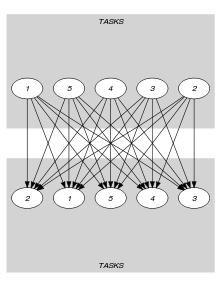(a)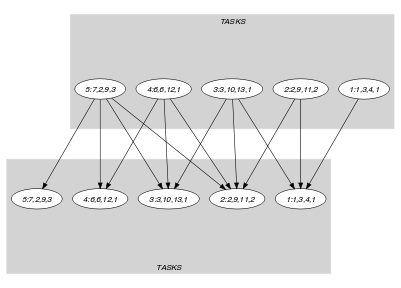(b)
Signature

Since $\mathrm{𝚃𝙰𝚂𝙺𝚂}$ is the maximum number of vertices of the final graph of the first graph constraint we can rewrite $\mathrm{𝐍𝐀𝐑𝐂}$ $=$ $|\mathrm{𝚃𝙰𝚂𝙺𝚂}|$ to $\mathrm{𝐍𝐀𝐑𝐂}$ $\ge$ $|\mathrm{𝚃𝙰𝚂𝙺𝚂}|$. This leads to simplify $\underline{\overline{\mathrm{𝐍𝐀𝐑𝐂}}}$ to $\overline{\mathrm{𝐍𝐀𝐑𝐂}}$.

Automaton

Figure 5.96.4 depicts the automaton associated with the $\mathrm{𝚌𝚞𝚖𝚞𝚕𝚊𝚝𝚒𝚟𝚎}$ constraint. To each item of the collection $\mathrm{𝚃𝙰𝚂𝙺𝚂}$ corresponds a signature variable ${S}_{i}$ that is equal to 1.

##### Figure 5.96.4. Automaton of the $\mathrm{𝚌𝚞𝚖𝚞𝚕𝚊𝚝𝚒𝚟𝚎}$ constraint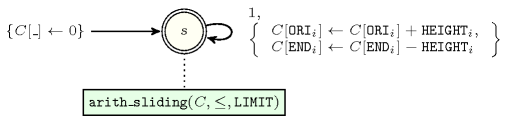Quiz

$\mathrm{𝚌𝚞𝚖𝚞𝚕𝚊𝚝𝚒𝚟𝚎}$: checking whether a ground instance holds or not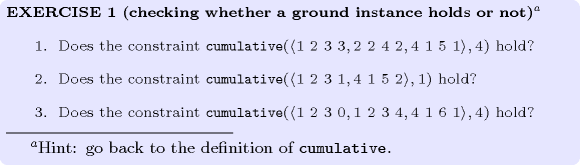$\mathrm{𝚌𝚞𝚖𝚞𝚕𝚊𝚝𝚒𝚟𝚎}$: finding all solutions